# Essential Discrete Mathematics for Computer Science

A more intuitive approach to the mathematical foundation of computer science Discrete mathematics is the basis of much of computer science, from algorithms and automata theory to combinatorics and graph theory. This textbook covers the discrete mathematics that every computer science student needs to learn. Guiding students quickly through thirty-one short chapters that dis A more intuitive approach to the mathematical foundation of computer science Discrete mathematics is the basis of much of computer science, from algorithms and automata theory to combinatorics and graph theory. This textbook covers the discrete mathematics that every computer science student needs to learn. Guiding students quickly through thirty-one short chapters that discuss one major topic each, this flexible book can be tailored to fit the syllabi for a variety of courses. Proven in the classroom, Essential Discrete Mathematics for Computer Science aims to teach mathematical reasoning as well as concepts and skills by stressing the art of proof. It is fully illustrated in color, and each chapter includes a concise summary as well as a set of exercises. The text requires only precalculus, and where calculus is needed, a quick summary of the basic facts is provided. Essential Discrete Mathematics for Computer Science is the ideal introductory textbook for standard undergraduate courses, and is also suitable for high school courses, distance education for adult learners, and self-study. The essential introduction to discrete mathematics Features thirty-one short chapters, each suitable for a single class lesson Includes more than 300 exercises Almost every formula and theorem proved in full Breadth of content makes the book adaptable to a variety of courses Each chapter includes a concise summary Solutions manual available to instructors

Compare

A more intuitive approach to the mathematical foundation of computer science Discrete mathematics is the basis of much of computer science, from algorithms and automata theory to combinatorics and graph theory. This textbook covers the discrete mathematics that every computer science student needs to learn. Guiding students quickly through thirty-one short chapters that dis A more intuitive approach to the mathematical foundation of computer science Discrete mathematics is the basis of much of computer science, from algorithms and automata theory to combinatorics and graph theory. This textbook covers the discrete mathematics that every computer science student needs to learn. Guiding students quickly through thirty-one short chapters that discuss one major topic each, this flexible book can be tailored to fit the syllabi for a variety of courses. Proven in the classroom, Essential Discrete Mathematics for Computer Science aims to teach mathematical reasoning as well as concepts and skills by stressing the art of proof. It is fully illustrated in color, and each chapter includes a concise summary as well as a set of exercises. The text requires only precalculus, and where calculus is needed, a quick summary of the basic facts is provided. Essential Discrete Mathematics for Computer Science is the ideal introductory textbook for standard undergraduate courses, and is also suitable for high school courses, distance education for adult learners, and self-study. The essential introduction to discrete mathematics Features thirty-one short chapters, each suitable for a single class lesson Includes more than 300 exercises Almost every formula and theorem proved in full Breadth of content makes the book adaptable to a variety of courses Each chapter includes a concise summary Solutions manual available to instructors

## 30 review for Essential Discrete Mathematics for Computer Science

1.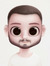4 out of 5

Erik Terres

2.4 out of 5

Jovany Agathe

3.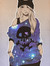4 out of 5

polluxhoe

4.5 out of 5

☘Misericordia☘ ⚡ϟ⚡⛈⚡☁ ❇️❤❣

5.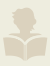4 out of 5

Robert Derricotte

6.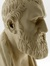5 out of 5

Alex

7.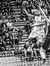5 out of 5

8.4 out of 5

Viet Trinh

9.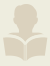5 out of 5

Matt Webster

10.4 out of 5

rev

11.5 out of 5

Jimmm

12.5 out of 5

Piotr Lis

13.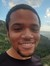5 out of 5

Stephen Fox

14.4 out of 5

Haksell

15.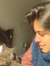5 out of 5

Sara Sampaio

16.5 out of 5

17.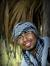5 out of 5

Hiren Gogoi

18.5 out of 5

Chukson

19.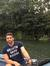4 out of 5

Moataz Elmasry

20.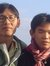5 out of 5

Surachai Lim

21.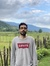5 out of 5

Pouya Pournasir

22.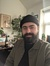4 out of 5

Josh Pannell

23.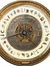4 out of 5

Nathan Jensen

24.5 out of 5

Nitish

25.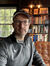4 out of 5

26.4 out of 5

Pokpong Chirayukool

27.4 out of 5

Hamio

28.5 out of 5

Azzaz Akl

29.5 out of 5

Avery Mensch

30.5 out of 5

∃Ⓐ∀